Learning Library

# Double Dose! Two Digit Multiplication for 3rd Grade

Looking for two digit multiplication practice for your third grader? Use this set of worksheets to support your child in building strong math skills.### Step by Step: Two-Digit Multiplication

With clear, step-by-step instructions, this worksheet is great for kids who haven't quite mastered their 10, 11 and 12 times tables.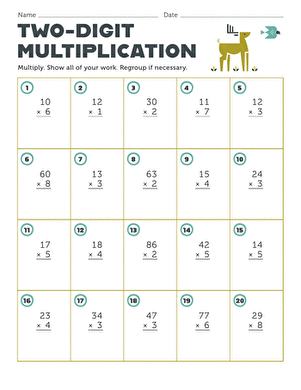### Two-Digit Multiplication

Build your child's two-digit multiplication skills with this worksheet.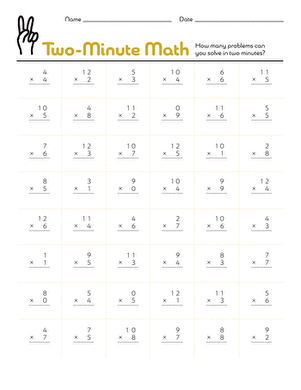### 2-Minute Multiplication

Do some mad multiplication with this heart-racing math minute worksheet! Math minutes bring out your child's competitive spirit in the best way.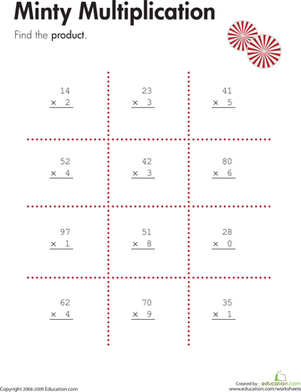### Two-Digit by One-Digit Multiplication

Kids solve problems involving multiplication of two-digit by one-digit numbers on this third grade math worksheet.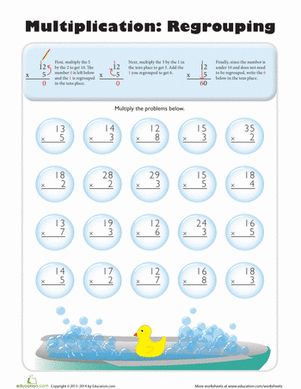### Multiplication Regrouping

This worksheet is jam-packed with 2 pages of multiplication regrouping problems to help kids tackle two-digit multiplication.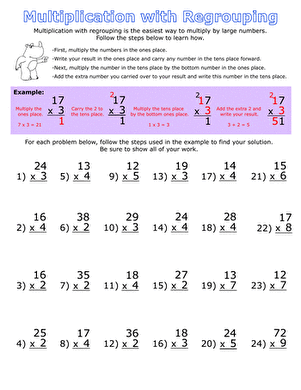### Multiplication with Regrouping

Offering over 40 regrouping problems, this worksheet is a great way to practice your multiplication skills.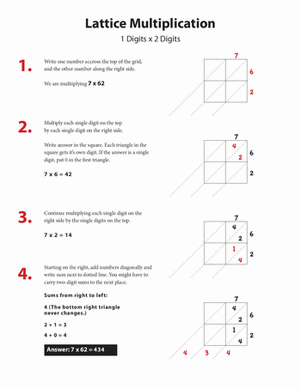### How To Do Lattice Multiplication

In third grade your child will be learning different methods of multiplying double digit numbers. The lattice method can be tricky but this guide will help.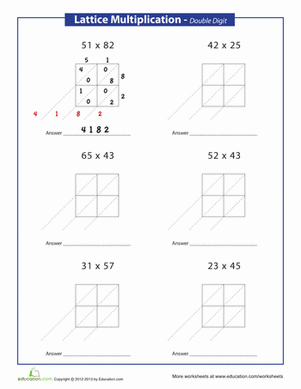### Lattice Method For Multiplication

The lattice method for multiplication may be a different way of multiplying than your child is used to, and could help practice multiplying double digits.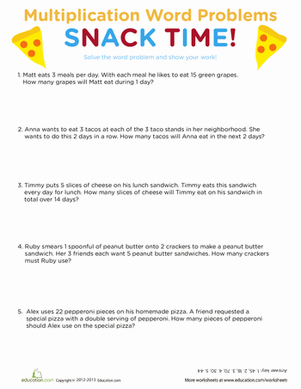### Snack Time Multiplication

Indulge in some delicious multiplication with this word problem worksheet that helps kids practice times tables and double-digit multiplication skills.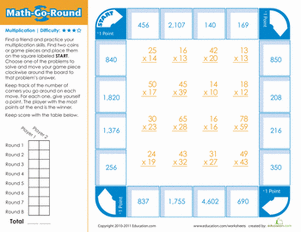### Math-Go-Round: Hard

Here's a fun board game that's sure to take the blahs out of multiplication practice.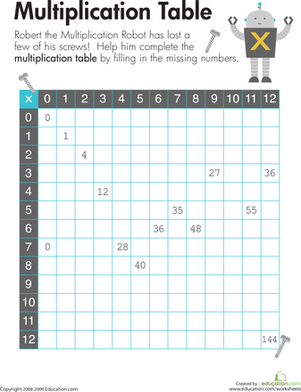### Multiplication Table 1-12

Kids fill in the missing spaces of the multiplication table for numbers 1 to 12 to complete this third grade math worksheet.

Create new collection

0

### New Collection>

0 items

What could we do to improve Education.com?